# Etale topology

(diff) ← Older revision | Latest revision (diff) | Newer revision → (diff)

The most important example of a Grothendieck topology (see Topologized category), making it possible to define cohomology and homotopy invariants for abstract algebraic varieties and schemes. Letbe a scheme. The étale topology onis the name for the category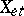of étale-schemes the objects of which are étale morphisms (cf. Etale morphism)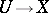and the morphisms of which are those of the-schemes. Finite familiessuch that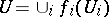are taken as coverings and so ina topology is introduced.

A pre-sheaf of sets (groups, Abelian groups, etc.) onis defined as a contravariant functor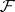from the categoryinto that of sets (groups, etc.). A pre-sheafis called a sheaf if for any covering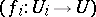a sectionis determined by its restriction to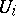and if for any compatible collection of sections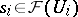there exists a unique section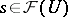such that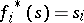. Many standard concepts of sheaf theory carry over to étale sheaves (that is, sheaves on). For example, ifis a morphism of schemes andis an étale sheaf on, then by puttingone obtains the so-called direct image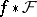offor the morphism. The functor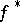adjoint to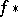on the left is called the inverse-image functor. In particular, the stalk ofat a geometric point(whereis an algebraically closed field) is defined as the set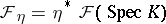.

An important example of a sheaf onis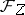, representable by a certain-scheme; for it. If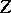is a finite étale-scheme, then the sheafis called locally constant. A sheafis said to be constructible if there exists a finite partition ofinto locally closed subschemessuch that the restriction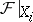is locally constant on every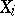.